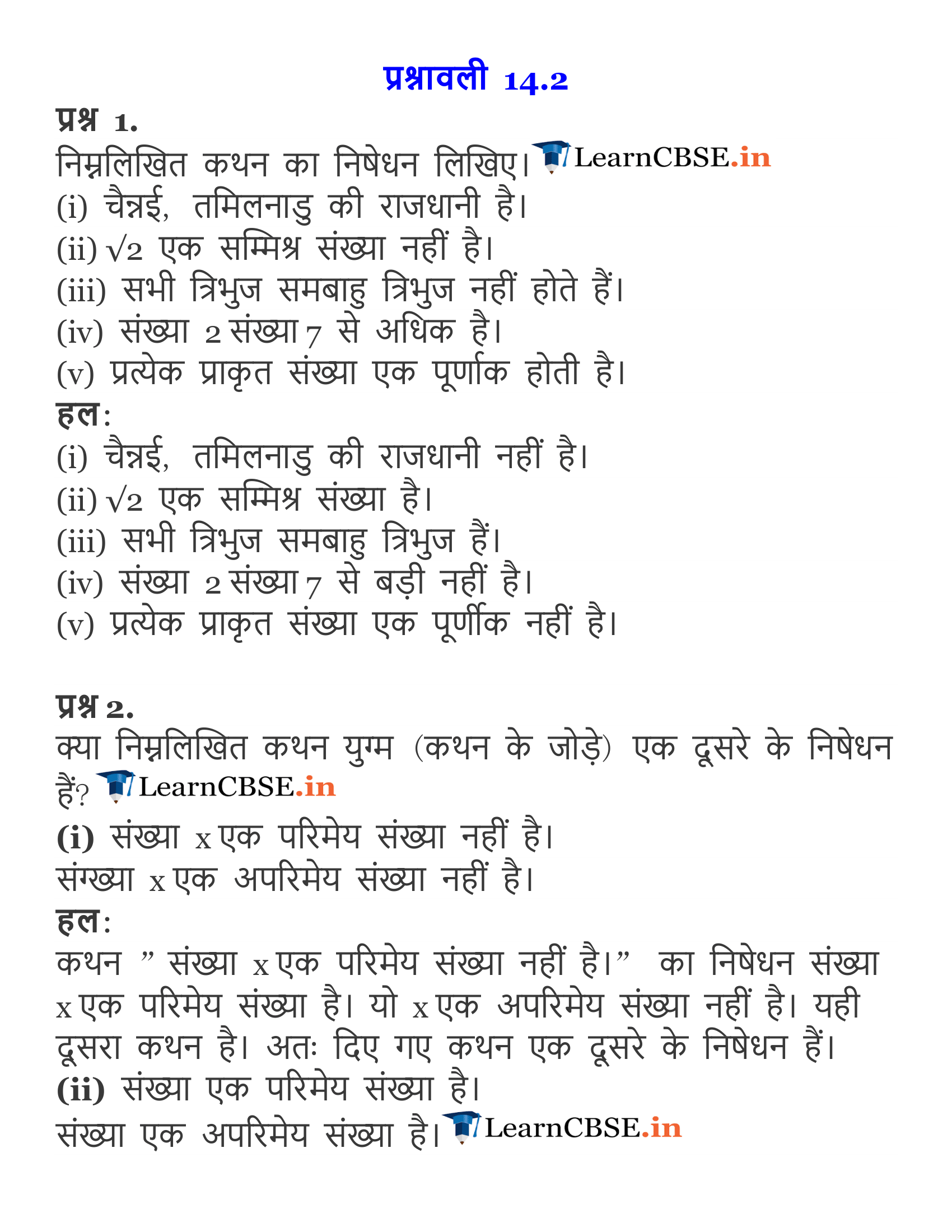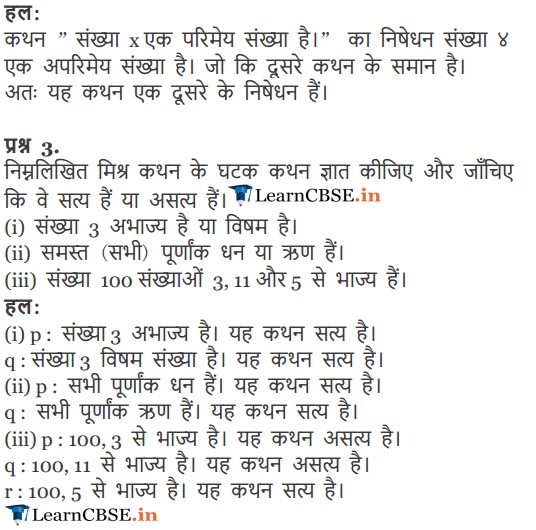Get Free NCERT Solutions for Class 11 Maths Chapter 14 Mathematical Reasoning Ex 14.2 PDF in Hindi and English Medium. Mathematical Reasoning Class 11 Maths NCERT Solutions are extremely helpful while doing your homework. Mathematical Reasoning Ex 14.2 Class 11 Maths NCERT Solutions were prepared by Experienced LearnCBSE.in Teachers. Detailed answers of all the questions in Chapter 14 Class 11 Mathematical Reasoning Ex 14.2 provided in NCERT Textbook.

Free download NCERT Solutions for Class 11 Maths Chapter 14 Mathematical Reasoning Ex 14.2 PDF in Hindi Medium as well as in English Medium for CBSE, Uttarakhand, Bihar, MP Board, Gujarat Board, BIE, Intermediate and UP Board students, who are using NCERT Books based on updated CBSE Syllabus for the session 2019-20.

## NCERT Solutions for Class 11 Maths Chapter 14 Ex 14.2

### NCERT Solutions for Class 11 Maths Chapter 14 Exercise.14.2

Question 1.
Write the negation of the following statements:
(i) Chennai is the capital of Tamil Nadu.
(ii) $$\sqrt { 2 }$$ is not a complex number.
(iii) All triangles are not equilateral triangle.
(iv) The number 2 is greater than 7.
(v) Every natural number is an integer.
Solution:
(i) Negation of statement is: Chennai is not the capital of Tamil Nadu.
(ii) Negation of statement is: $$\sqrt { 2 }$$ is a complex number.
(iii) Negation of statement is: All triangles are equilateral triangles.
(iv) Negation of statement is: The number 2 is not greater than 7.
(v) Negation of statement is: Every natural number is not an integer.

Question 2.
Are the following pairs of statements negations of each other:
(i) The number x is not a rational number.
The number x is not an irrational number.
(ii) The number x is a rational number.
The number x is an irrational number.
Solution:
(i) Let p: The number x is not a rational number.
q: The number x is not an irrational number.
Now, ~p: The number x is a rational number. ~q: The number x is an irrational number.
∴ ~p = q and ~q = p
Thus, p and q are negations of each other.

(ii) Let p: The number x is a rational number.
q: The number x is an irrational number.
Now, ~p: The number x is not a rational number.
~q: The number x is not an irrational number.
∴ ~p = q and ~q = p
Thus, p and q are negations of each other.

Question 3.
Find the component statements of the following compound statements and check whether they are true or false.
(i) Number 3 is prime or it is odd.
(ii) All integers are positive or negative.
(iii) 100 is divisible by 3,11 and 5.
Solution:
(i) The component statements are:
p: Number 3 is prime
q: Number 3 is odd.
Both the component statements p and q are true.

(ii) The component statements are:
p: All integers are positive.
q: All integers are negative.
Both the component statements p and q are false.

(iii) The component statements are:
p: 100 is divisible by 3.
q: 100 is divisible by 11.
r: 100 is divisible by 5.
The component statements p and q are false whereas r is true.

### NCERT Solutions for Class 11 Maths Chapter 14 Mathematical Reasoning (गणितीय विवेचन) Hindi Medium Ex 14.2